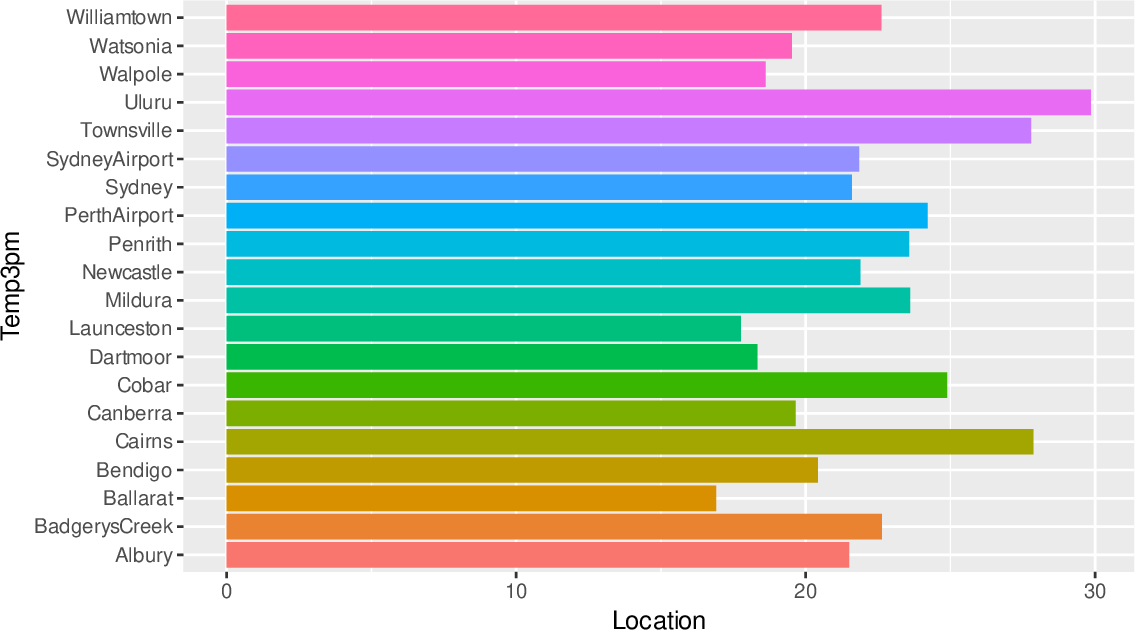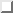Data Science Desktop Survival Guide by Graham WilliamsDesktop Survival Project Home Preface Data Science Introducing R R Constructs R Tasks R Strings R Read, Write, and Create Data Template Data Exploration Data Wrangling Data Visualisation Statistics ML Template ML Scenarios ML Activities ML Applications ML Algorithms Cluster Analysis Decision Trees Computer Vision Graph Data Privacy Literate Data Science Coding with Style Resources Bibliography Index

## Flipped Bar Chart

20200608ds %>%   filter(location %in% (ds\$location %>% unique %>% sample(20))) %>%   ggplot(aes(location, temp_3pm, fill=location)) +   stat_summary(fun="mean", geom="bar") +   theme(legend.position="none") +   labs(x=vnames["temp_3pm"], y=vnames["location"]) +   coord_flip() We can flip the coordinates and produce a horizontal histogram as in Figure. Note that for clarity of presentation here we have also reduced the number of locations but would note that for an actual data science report we would include full plots. Rotating the plot is a more useful solution than simply rotating the labels as we can then add more bars down the page than we might across the page and it is easier for us to read the labels left to right rather than bottom up.